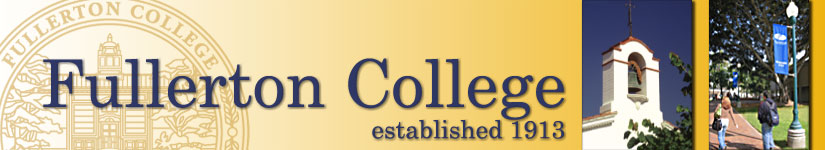## computer science courses

CSCI 123 F: Introduction to Programming Concepts in C++ (4 units) (F,S)
Prerequisites: Math 141 College Algebra or Math 142 Trigonometry or three years of college preparatory high school mathematics including trigonometry with grades of "C" or better. Reading 96 level competency strongly advised.

Four hours lecture and one hour arranged per week. This course is an introduction to the basic principles of programming using C++ as the development tool. Topics include the structure and design of algorithms, input/output, branching structures, functions, recursion, built-in data types, arrays, structures, files, pointers and elementary operations on linked structures. The object-oriented programming paradigm will be introduced. Topics include encapsulation, polymorphism, libraries, stream, inheritance and abstract data types. Students will design algorithms, write external and internal documentation and design and write source code in C++. (CSU) (UC) (Degree Credit)

CSCI 133 F: Data Structures in C++ (4 units) (F,S)
Prerequisite: CSCI 123 Introduction to Programming Concepts in C++ or equivalent with a grade of "C" or better.

Four hours lecture and one hour arranged per week. This is a course in algorithm design and data structures implemented using C++ classes. Structures studied are arrays, linked lists, stacks, queues, trees, tables, and graphs. Algorithm topics studied include hashing, sorting, heaps, searches and algorithm efficiency using Big-O notation. (CSU) (UC) (Degree Credit)

CSCI 223 F: C Language for Mathematics and Science (4 units) CAN CSCI 16 (F,S)
Prerequisites: CSCI 123 Introduction to Programming Concepts in C++ or equivalent with a grade of "C" or better.

Four hours lecture and one hour arranged per week. This course is an introduction to the C programming language. One of the latest C compilers will be used on a personal computer. Topics include data types, functions, pointers, bit manipulation and file I/O. Students will design, code and test program applications in the mathematics, scientific and engineering environments.. (CSU) (UC) (Degree Credit)

CSCI 241 F: Assembly Language Programming (4 Units) (S)
Prerequisite: CSCI 133 Data Structures in C++ or CSCI 223 C Programming for Mathematics and Science with a grade of "C" or better.

Four lecture hours and one hour arranged per week. This course is an introduction to assembly language programming. It includes reviews of computer organization, programming techniques and concepts, addressing techniques, input/output, hardware architecture, and data structures. (CSU) (UC credit limitation) (Degree Credit)

## related math courses

MATH 171 F Discrete Mathematics (4)

Prerequisite: MATH 141 F and MATH 142 F, both with a grade of “C” or better, or equivalent, or math skills clearance

Four lecture hours per week. This is the first of two semesters of fundamental discrete mathematical concepts needed in computer related disciplines. The topics covered include logic, truth tables, Boolean algebra, logic circuits, elementary set theory, functions, relations, proof techniques, combinatorics, elementary probability and recurrence relations. (CSU) (UC) (Degree Credit) AA GE, CSU GE, IGETC

MATH 172 F Graph Theory and Linear Algebra (4)

Prerequisite: MATH 171 F or equivalent with a grade of “C” or better
Four hours lecture per week. This is the second of two semesters of fundamental discrete mathematical concepts and techniques needed in computer related disciplines. Topics include the theory of graphs, trees, finite state machines, and linear algebra including matrix operations, eigenvalues, vector spaces, linear transformations, and inner product spaces. (Degree Credit) (CSU) (UC) AA GE, CSU GE, IGETC

To reach the Math Division, please click here.